# SAT Math Multiple Choice Question 267: Answer and Explanation

### Test Information

Question: 267

12.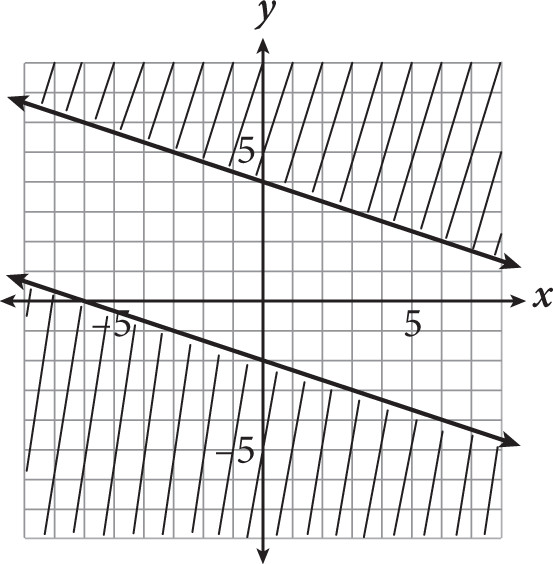Which of the following systems of inequalities could be represented by the graph shown?

• A.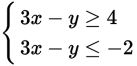• B.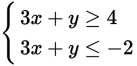• C.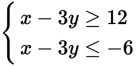• D.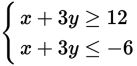Getting to the Answer: The equations are all given in standard form (Ax + By = C). It would be very time-intensive to convert all four systems to slope-intercept form, so look for ways to eliminate choices more quickly. Each line in the graph falls 1 unit and runs 3 units, so you need to find two lines that have a slope of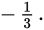Use the trick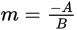to quickly determine the slopes. The lines in A have a slope of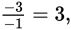so eliminate A; the lines in B have a slope of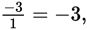so eliminate B; the lines in C have a slope of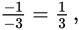so eliminate C (pay attention to the sign). This means (D) must be correct. The lines in (D) have a slope of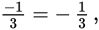which matches the graph. You don't have to check the shading because none of the other slopes were a match.